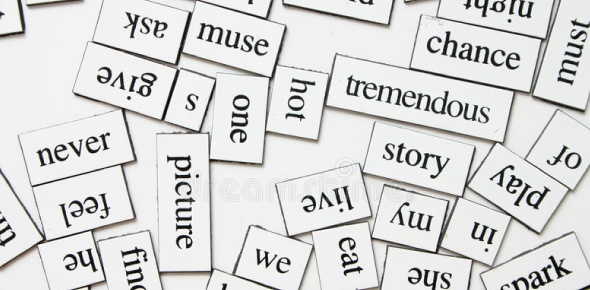# Stems 7 Quiz

25 Questions | Total Attempts: 294Settings.

Related Topics
• 1.
Numer =
• 2.
Fort =
• 3.
Osteo =
• 4.
Polis =
• 5.
Ornith =
• 6.
Fus =
• 7.
Ego =
• 8.
Spir =
• 9.
Dia =
• 10.
Acr =
• 11.
Acro =
• 12.
Culp =
• 13.
Derm =
• 14.
Zo =
• 15.
Per =
• 16.
Pac =
• 17.
Brev =
• 18.
Necro =
• 19.
Urb =
• 20.
Pugn =
• 21.
Ecto =
• 22.
Plasto =
• 23.
Agog =
• 24.
Cle =
• 25.
Il =